Courses

# Surveying (Part - 10) Civil Engineering (CE) Notes | EduRev

## Civil Engineering (CE) : Surveying (Part - 10) Civil Engineering (CE) Notes | EduRev

The document Surveying (Part - 10) Civil Engineering (CE) Notes | EduRev is a part of the Civil Engineering (CE) Course Civil Engineering SSC JE (Technical).
All you need of Civil Engineering (CE) at this link: Civil Engineering (CE)

Surveying (Part 10)

METHOD OF CURVE RANGE :
1. Linear or chain and tape method and 2. Angular or instrumental methods. 

• Linear methods is those in which the curve is set out with a chain and tape only.  Instrumental methods are those in which a theodolite with or without a chain is employed to set out the curve.

PEG INTERVAL.
The length of unit chord (peg interval) is, therefore, 30 m for flat curve 20 m for sharp curves and 10m or less for very sharp curves.

LOCATION OF TANGENT POINTS:
To locate the tangent points T1 and T2, proceeds as follows:

(i) Having fixed the direction of the tangents, produce them so as to meet at the point B
(ii) Find the deflection angle by theodolite.
(iii) Calculate the tangent length BT1 = BT2 = R tan OB = R sec f/2

(iv) Locate the point T1 and T2 on back tangent and forward tangent.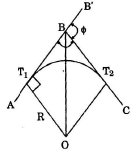� Chainage of point T1 = Chainage of point of intersection B – BT1 Chainage of point T2 = chainage of point T1 + length of the curve Chain and Tape (or linear) methods  of setting out curves

1. By offsets or ordinates from the long chord (Linear method)
2. Offsets from the tangents (Linear method)
3. Successive bisection of the chords (linear method)

Angular or Instrumental Methods
1. Offsets from Chord produced (Linear method)
2. Deflection angles from the point of curve and normal chord (Angular method)
3. Deflection angles from the point of cure and point of tangency, using two theodolite (Angular method)

1. Offsets from the Long Chord :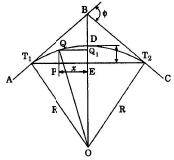Let AB and BC = tangents to the curve T1DT2 T1 and Tar tangent points
T1 T2 = Long chord of length L.
ED = O= offset at the mildpoint of
T1T2 (the versed sine) PQ = Qx = offset at a distance x from E, so that
EP = x OT1 = OT2 = OD = R radius of the curve

In DOT1E, OT = R, T1E = L/2 OT = OD -ED = R-O0

OT12 = T1E2+ OE2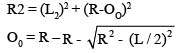In DOQQ1 OQ= QQ12 + OQ12

� R2 = x2 + (OE + Ox)
� OE + Ox =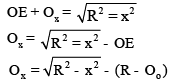[since OE = OD – ED = R-O]

2. OFFSET FROM THE TANGENTS :
(i) Perpendiculr offsets

(i) Perpendicular off sets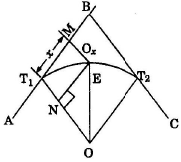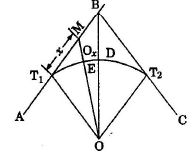DE2 = NE2 + ON2
� R2 = x2 + (R-Ox)3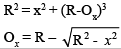3. SUCCESSIVE BISECTION OF CHORDS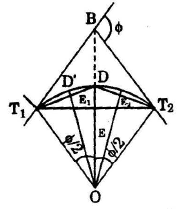Let T1T2 be the long chord of a curve whose angle of deflection is f.
Divide T1T2 at C. Joint OE and produce it to intersect the curve at Now DE = OD-OE = R-R cos f/2 Oo = DE = R (1-cos f/2) Now consider T1D and T2D independent portions of the curve having T1D and T2D as long chord. it can be proved that offset E1D1 and E2D2 are each equal to R (1–cos f/4).
By further successive bisection of the chords T1D1, D1D1, DD2 and D2T2 we may obtain the locations of other points on the curve.

4. OFFSET FROM CHORD PRODUCED:
This method is commonly adopted when a theodolie is not available and it is necessary to set out a curve only with a chain or a tape. The curve is divided into a number of chords normally 20 or 30 m in length. As continuous chainage is required along the curve, two sub-chords generally occurs one at the beginning and the other at the end of the curve.
Offsets from chords produced may be computed with the help of the formula derived under :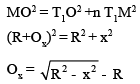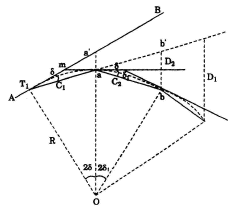Let AB be the back tangent, T1a = C1 be the first sub-chord and � BT1a =d OT1 = Oa = R The chord T1a being very newly equal to are T1a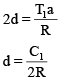�...............(i)

Similarly the chord aa’ is very neurly equal to the arc aa’

or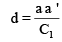� a a ' =dC1 .............. (ii)
From equations (i) and (ii)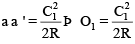.............. (iii)

Now mT1 and ma both being tangents � mT1a = � maT1 =d = � b' an (opposite angles)

Now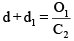.............(iv)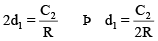..............(v)

Putting the value of d and d1 in equation (iii)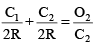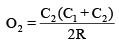..............(vi)

Similary we get the value of third offset O3 i.e, O3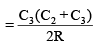All the chords, excepting the sub-chords are generally equal .............(vii)

i.e. C2 = C3 = C4 = ----------Cn-1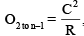where C is the length of the normal chord

The offsets for the last sub chord Cn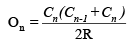5. Ranking Method of Trngential Deflection Angles: 

Offer running on EduRev: Apply code STAYHOME200 to get INR 200 off on our premium plan EduRev Infinity!

## Civil Engineering SSC JE (Technical)

109 docs|50 tests

,

,

,

,

,

,

,

,

,

,

,

,

,

,

,

,

,

,

,

,

,

;